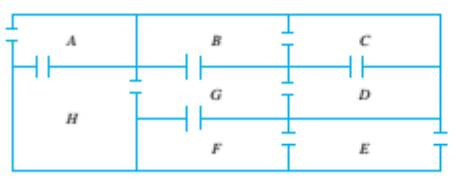Chapter 10.1, Problem 22ES### Discrete Mathematics With Applicat...

5th Edition
EPP + 1 other
ISBN: 9781337694193

#### Solutions

Chapter
Section### Discrete Mathematics With Applicat...

5th Edition
EPP + 1 other
ISBN: 9781337694193
Textbook Problem
1 views

# The following is a floor plan of a house. Is it possible to enter the house in room A, travel through every interior doorway of the house exactly once, and exit out of room E? If so, how can this be doneTo determine

Is it possible to enter the house in room A, travel through every interior doorway of the house exactly once, and exit out of room E ? If so, how can this be done?

Explanation

Given information:

The following is a floor plan of a house.

Calculation:

We reduce the problem graphically

The given problem is equivalent to finding an Euler path from A to E...

### Still sussing out bartleby?

Check out a sample textbook solution.

See a sample solution

#### The Solution to Your Study Problems

Bartleby provides explanations to thousands of textbook problems written by our experts, many with advanced degrees!

Get Started